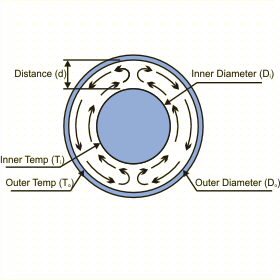This page calculates the natural convection heat transfer between two concentric cylinders maintained at constant temperatures. The calculation is based on Rayleigh number and is valid for Rayleigh numbers below 107.  Define the dimensions of the cylinders and their temperatures. You also need to define the fluid properties. This page will calculate the heat convected between the cylinders based on the flow conditions.Do you want to know more ?Cylinders and Flow Conditions

Fluid Properties at Film Temperature (Default is for Air at 20C)

 Description Symbol Value Units Film Temperature = (Ti+To)/2 Tf oC Specific Heat Cp J/kg-oC Coefficient of Thermal Expansion B 1/K Thermal Conductivity k W/m-oC Dynamic Viscosity Á kg/m-s Density at reference temperature of 20 C rhoref kg/m3 Density calculated at film temperature Tf rho kg/m3

Cylinders Dimensions

 Description Symbol Value Units Length L Meters Inner Cylinder Diameter Di Meters Outer Cylinder Diameter Do Meters Inner Cylinder Temperature Ti oC Outer Cylinder Temperature To oC

Results for Concentric Cylinders

 Description Symbol Value Units Gap between cylinders d m Effective conductivity keff W/m-oC Prandlt Number = Cp*Á / k Pr None Rayleighd = gB rho2Cp(Ti-To)d3 / kÁ Rad None Rayleighc* = Rad (ln(Do/Di))4) / d3(Di-3/5+Do-3/5)5 Rac* None Heat transfer = 2Pi keff L (Ti-To) / ln(Do/Di) q W
 Copyright and Disclaimer Noticepowered by MAYA HTT